NCERT Class 7 Maths Algebraic Expressions

# NCERT Class 7 Maths Algebraic Expressions

The chapter 12 begins with an introduction to Algebraic Expressions by citing some examples of algebraic expressions in one variable. Then the concept of how expressions are formed and terms of an expression are explained.Under this, the factors of a term and coefficients are discussed in detail. Then we have the discussion of like and unlike terms.Monomials, Binomials, Trinomials and Polynomials are described in the next section.Addition and subtraction of algebraic expressions is explained in the following section. Then, we have the procedure to finding the value of an expression. The last topic of the chapter is formulas and rules in mathematics written in a concise and general form using algebraic expressions.

Download FREE PDF of Chapter-12 Algebraic Expressions

## Chapter 12 Ex.12.1 Question 1

Get the algebraic expressions in the following cases using variables, constants and arithmetic operations.

(i) Subtraction of $$z$$ from $$y.$$

(ii) One-half of the sum of numbers $$x$$ and $$y.$$

(iii) The number $$z$$ multiplied by itself.

(iv) One-fourth of the product of numbers $$p$$ and $$q.$$

(v) Numbers $$x$$ and $$y$$ both squared and added.

(vi) Number $$5$$ added to three times the product of numbers $$m$$ and $$n.$$

(vii) Product of numbers $$y$$ and $$z$$ subtracted from $$10.$$

(viii) Sum of numbers $$a$$ and $$b$$ subtracted from their product.

### Solution

Reasoning:

Let us first understand the meaning or definition of terms variable, constants and arithmetic operations

Variables are the letters used in an algebraic expression that can take any value. For e.g. $$a, b, c$$ or $$z$$ etc. and it can take any value which can be either $$2$$ or $$5$$ or any other number. Constants always have fixed values in the algebraic expressions. They cannot be assumed or changed. Arithmetic Operations are Addition, subtraction, multiplication and division.

Steps:

(i) Subtraction of $$z$$ from $$y.$$

$y - z$

(ii) One-half of the sum of numbers $$x$$ and $$y.$$

$\frac{1}{2}\left( {x + y} \right)$

(iii) The number $$z$$ multiplied by itself.

$z \times z = {z^2}$

(iv) One-fourth of the product of numbers $$p$$ and $$q.$$

$\frac{1}{4}pq$

(v) Numbers $$x$$ and $$y$$ both squared and added.

$\left( {x \times x} \right) + \left( {y \times y} \right) = {x^2} + {y^2}$

(vi) Number $$5$$ added to three times the product of numbers $$m$$ and $$n.$$

$5 + 3\left( {m \times n} \right) = 5 + 3mn$

(vii) Product of numbers $$y$$ and $$z$$ subtracted from $$10.$$

$10 - \left( {y \times z} \right) = 10 - yz$

(viii) Sum of numbers $$a$$ and $$b$$ subtracted from their product.

$\left( {a \times b} \right)-\left( {a + b} \right) = ab - \left( {a + b} \right)$

## Chapter 12 Ex.12.1 Question 2

(i) Identify the terms and their factors in the following expressions. Show the terms and factors by tree diagrams.

(a) $$x – 3$$

(b) $$1 + x + x^2$$

(c) $$y – y^3$$

(d) $$5xy^2 + 7x^2y$$

(e) $$– ab + 2b^2 – 3a^2$$

(ii) Identify terms and factors in the expressions given below:

(f) $$–4x + 5$$ (b) $$–4x + 5y$$ (c) $$5y + 3y^2$$

(g) $$xy + 2x^2y^2$$ (e) $$pq + q$$ (f) $$1.2 ab – 2.4 b + 3.6 a$$

(h) $$\frac{3}{4}x + \frac{1}{4}$$ (h) $$0.1 p^2 + 0.2 q^2$$

### Solution

(i) Identify the terms and their factors in the following expressions. Show the terms and factors by tree diagrams.

(a) $$x – 3$$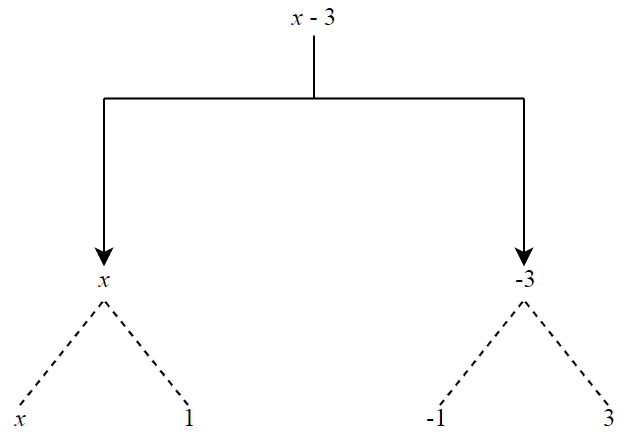Steps:

Term $$= x$$ and Factor $$= 1$$

(b) $$1 + x + x^2$$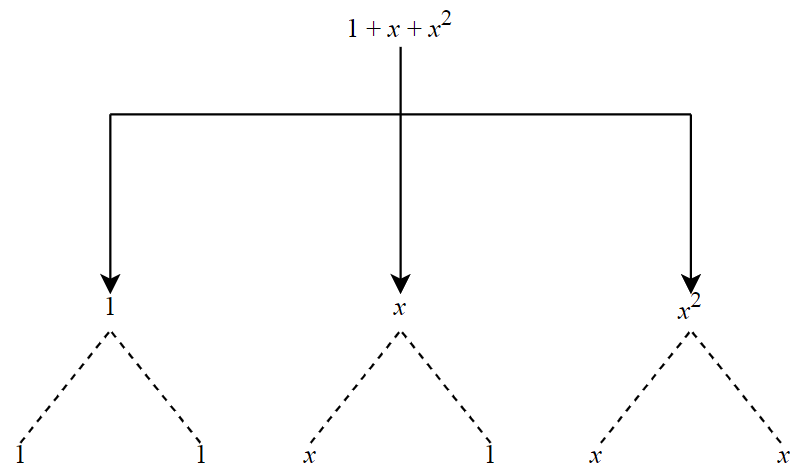Steps:

Term $$= x$$ and Factor $$= 1;$$ Term $$= x^2$$ and Factor $$= 1$$

(c) $$y – y^3$$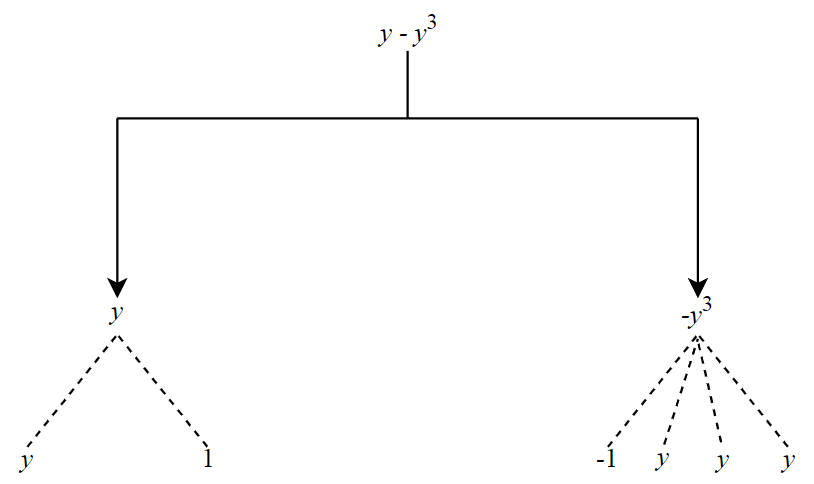Steps:

Term $$= y$$ and Factor $$= 1;$$ Term $$= y^3$$ and Factor $$= –1$$

(d) $$5xy^2 + 7x^2y$$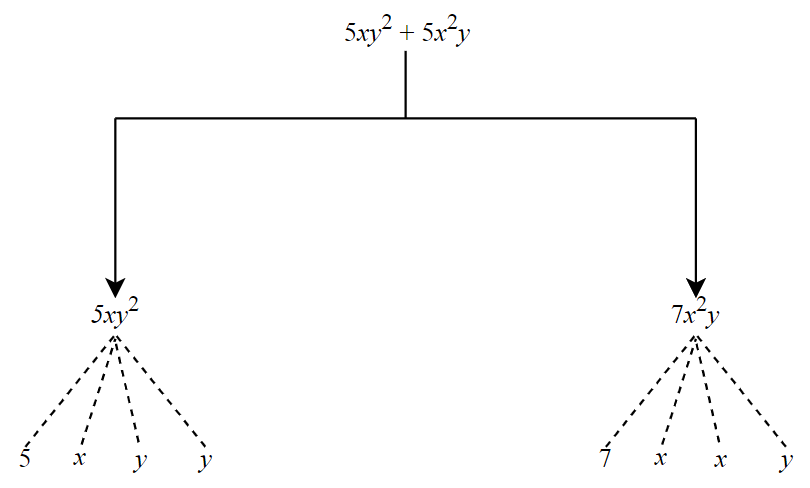Steps:

Term $$= xy^2$$ and Factor $$= 5;$$ Term $$= x^2y$$ and Factor $$= 7$$

(e) $$– ab + 2b^2 – 3a^2$$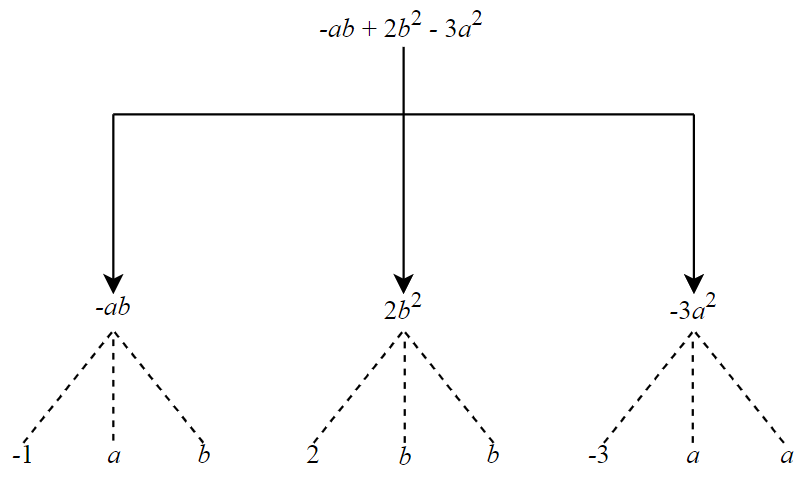Steps:

Term $$= ab$$ and Factor $$= –1;$$Term $$= b^2$$ and Factor $$= 2;$$ Term $$a^2$$ and Factor $$= –3$$

(ii) Identify terms and factors in the expressions given below:

(f) $$–4x + 5$$ (b) $$–4x + 5y$$ (c) $$5y + 3y^2$$

(g) $$xy + 2x^2y^2$$ (e) $$pq + q$$ (f) $$1.2 ab – 2.4 b + 3.6 a$$

(h) $$\frac{3}{4}x + \frac{1}{4}$$ (h) $$0.1 p^2 + 0.2 q^2$$

 S.No. Expression Term Factors a) $$–4x + 5$$ $$-4x$$ and $$5$$ $$-4, x$$and $$5$$ b) $$-4x + 5y$$ $$-4x$$ and $$5y$$ $$-4, x$$ and $$5, y$$ c) $$5y + 3y^2$$ $$5y$$ and $$3y^2$$ $$5, y$$ and $$3, y, y$$ d) $$xy + 2x^2y^2$$ $$xy$$ and $$2x^2y^2$$ $$x, y$$ and $$2, x, x,y, y$$ e) $$pq + q$$ $$pq$$ and $$q$$ $$p, q$$ and $$q$$ f) $$1.2ab - 2.4b + 3.6a$$ $$1.2ab, -2.4b$$ and $$3.6a$$ $$1.2, a, b, -2.4, b$$ and $$3.6, a$$ g) $$\frac{{3}}{4} x + \frac{{1}}{4}$$ $$\frac{{3}}{4} x$$ and $$\frac{{1}}{4}$$ $$\frac{{3}}{4}, x$$ and $$\frac{{1}}{4}$$ h) $$0.1 p^2 + 0.2 q^2$$ $$0.1p^2$$ and $$0.2q^2$$ $$0.1, p, p$$ and $$0.2, q, q$$

## Chapter 12 Ex.12.1 Question 3

Identify the numerical coefficients of terms (other than constants) in the following expressions:

(i) $$5 – 3t^2$$

ii) $$1 + t + t^2 + t^3$$

(iii) $$x + 2xy + 3y$$

(iv) $$100m + 1000n$$

(v) $$– p^2q^2 + 7pq$$

(vi) $$1.2a + 0.8b$$

(vii) $$3.14r^2$$

(viii) $$2(l + b)$$

(ix) $$0.1y + 0.01y^2$$

### Solution

 S.No. Expression Term Numerical Coefficient (i) $$5 - 3t^2$$ $$-3t^2$$ $$-3$$ (ii) $$1 + t + t^2 + t^3$$ $$t, t^2$$ and $$t^3$$ $$1, 1$$ and $$1$$ (iii) $$x + 2xy + 3y$$ $$x , 2xy$$ and $$3y$$ $$1, 2$$ and $$3$$ (iv) $$100m + 1000n$$ $$100m$$ and $$1000n$$ $$100$$ and $$1000$$ (v) $$-p^2q^2 + 7pq$$ $$-p^2q^2$$ and $$7pq$$ $$-1$$ and $$7$$ (vi) $$1.2 a + 0.8 b$$ $$1.2a$$ and $$0.8b$$ $$1.2$$ and $$0.8$$ (vii) $$3.14r^2$$ $$3.14r^2$$ $$3.14$$ (viii) $$2(l + b)$$ $$2l$$ and $$2b$$ $$2$$ and $$2$$ (ix) $$0.1y + 0.01y^2$$ $$0.1y$$ and $$0.01 y^2$$ $$0.1$$ and $$0.01$$

## Chapter 12 Ex.12.1 Question 4

(a) Identify terms which contain $$x$$ and give the coefficient of $$x.$$

(i) $$y^2x + y$$

(ii) $$13y^2 – 8yx$$

(iii) $$x + y + 2$$

(iv) $$5 + z + zx$$

(v) $$1 + x + xy$$

(vi) $$12xy^2 + 25$$

(vii) $$7x + xy^2$$

(b) Identify terms which contain $$y^2$$ and give the coefficient of $$y^2.$$

(i) $$8 – xy^2$$

(ii) $$5y^2 + 7x$$

(iii) $$2x^2y – 15xy^2$$ + $$7y^2$$

### Solution

(a) Identify terms which contain $$x$$ and give the coefficient of $$x.$$

(i) $$y^2x + y$$

(ii) $$13y^2 – 8yx$$

(iii) $$x + y + 2$$

(iv) $$5 + z + zx$$

(v) $$1 + x + xy$$

(vi) $$12xy^2 + 25$$

(vii) $$7x + xy^2$$

 S.No. Expression Term containing x Coefficient of x (i) $$y^2x + y$$ $$y^2x$$ $$y^2$$ (ii) $$13y^2 – 8yx$$ $$–8yx$$ $$–8y$$ (iii) $$x + y + 2$$ $$x$$ $$1$$ (iv) $$5 + z + zx$$ $$zx$$ $$z$$ (v) $$1 + x + xy$$ $$x$$ and $$xy$$ $$1$$ and $$y$$ (vi) $$12xy^2 + 25$$ $$12xy^2$$ $$12y^2$$ (vii) $$7 x + xy^2$$ $$7 x$$ and $$xy^2$$ $$7$$ and $$y^2$$

(b) Identify terms which contain $$y^2$$ and give the coefficient of $$y^2.$$

(i) $$8 – xy^2$$

(ii) $$5y^2 + 7x$$

(iii) $$2x^2y – 15xy^2$$ + $$7y^2$$

 S.No. Expression Term containing y2 Coefficient of y2 (i) $$8 – xy^2$$ $$– xy^2$$ $$– x$$ (ii) $$5y^2 + 7x$$ $$5y^2$$ $$5$$ (iii) $$2x^2 y – 15xy^2 + 7y^2$$ $$– 15xy^2$$ and $$7y^2$$ $$– 15x$$ and $$7$$

## Chapter 12 Ex.12.1 Question 5

Classify into monomials, binomials and trinomials.

(i) $$4y – 7z$$

(ii) $$y^2$$

(iii) $$x + y – xy$$

(iv) $$100$$

(v) $$ab – a – b$$

(vi) $$5 – 3t$$

(vii) $$4p^2q – 4pq^2$$

(viii) $$7mn$$

(ix) $$z^2 – 3z + 8$$

(x) $$a^2 + b^2$$

(xi) $$z^2 + z$$

(xii) $$1 + x + x^2$$

### Solution

Steps:

Monomial means expression having single term.

Binomials means expression having two terms.

Trinomials means expression having three terms.

 S No. Expression No. of terms Classification (i) $$4y – 7z$$ $$2$$ Binomial (ii) $$y^2$$ $$1$$ Monomial (iii) $$x + y – xy$$ $$3$$ Trinomial (iv) $$100$$ $$1$$ Monomial (v) $$ab – a – b$$ $$3$$ Trinomial (vi) $$5 – 3t$$ $$2$$ Binomial (vii) $$4p^2q – 4pq^ 2$$ $$2$$ Binomial (viii) $$7mn$$ $$1$$ Monomial (ix) $$z^2 – 3z + 8$$ $$3$$ Trinomial (x) $$a^2 + b^2$$ $$2$$ Binomial (xi) $$z^2 + z$$ $$2$$ Binomial (xii) $$1 + x + x^2$$ $$3$$ Trinomial

## Chapter 12 Ex.12.1 Question 6

State whether a given pair of terms is of like or unlike terms.

(i) $$1, 100$$

(ii) $$–7x, x$$

(iii) $$– 29x, – 29y$$

(iv) $$14xy, 42yx$$

(v) $$4m^2p, 4mp^2$$

(vi) $$12xz, 12x^2z^2$$

### Solution

 S.No. Expression Terms Factors Like/ Unlike Reason (i) $$1, 100$$ $$1$$ and $$100$$ $$1$$ and $$100$$ Like Bothe the terms has no variables (ii) $$–7x, x$$ $$–7x$$ and $$x$$ $$–7, x$$ and $$x$$ Like Both terms have same variable $$x$$ (iii) $$– 29x, – 29y$$ $$– 29x$$ and $$– 29y$$ $$– 29, x$$ and $$– 29, y$$ Unlike Both terms have different variables $$x$$ & $$y$$ (iv) $$14xy, 42yx$$ $$14xy$$ and $$42yx$$ $$14, x,y$$ and $$42, y,x$$ Like Both terms have same variable $$xy$$ & $$xy$$ (v) $$4m^2p, 4mp^2$$ $$4m^2p$$ and $$4mp^2$$ $$4, m^2, p$$ and $$4, m, p^2$$ Unlike Both terms have same variable but with different powers (vi) $$12xz, 12x^2z^2$$ $$12xz$$ and $$12x^2z^2$$ $$12, x ,z$$ and $$12,x^2 ,z^2$$ Unlike Both terms have same variable but with different powers

## Chapter 12 Ex.12.1 Question 7

Identify like terms in the following:

(a)

\begin{align}&–xy^2, –4yx^2, 8x^2, 2xy^2, 7y, –11x^2, \\&–100x, –11yx, 20x^2y, –6x^2, \\&y, 2xy, 3x\end{align}

(b)

\begin{align}&10pq, 7p, 8q, –p^2q^2, –7qp, –100q,\\& –23, 12q^2p^2,–5p^2, 41,2405p, \\& 78qp, 13p^2q, qp^2, 701p^2 \end{align}

### Solution

Reasoning:

This question is based on the concept of like terms. If there are same variable in all
the terms in the expression, then the expression has like terms. We have to ignore constants here.

Steps:

 S.No. Terms Like terms (i) $$–xy^2, –4yx^2, 8x^2, 2xy^2, 7y, –11x^2, \\–100x, – 11yx, 20x^2y, –6x^2, y, 2xy, 3x$$ $$–xy^2, 2xy^2;\\ –4yx^2, 20x^2y;\\ 8x^2, –11x^2, –6x^2;\\ 7y, y;\\ –100x, 3 x;\\ –11yx, 2xy\\$$ (ii) $$10pq, 7p, 8q, –p^2q^2, –7qp, –100q, –23, 12q^2p^2,\\ –5p^2,41, 2405p, 78qp, 13p^2q, qp^2, 701p^2$$ $$10pq, –7qp, 78qp;\\ 8q, –100q;\\ -5p^2, 701p^2;\\ 7p, 2405p;\\ –p^2q^2, 12q^2p^2;\\ -23, 41;\\ 13p^2q, qp^2\\$$
Algebraic Expressions | NCERT Solutions
Instant doubt clearing with Cuemath Advanced Math Program